# An Insurance Sales Prediction Model Based on Deep Learning

An Insurance Sales Prediction Model Based on Deep Learning
School of Insurance, Shandong University of Finance and Economics, Jinan 250014, China

Corresponding Author Email:
20030328@sdufe.edu.cn
Page:
315-321
|
DOI:
https://doi.org/10.18280/ria.340309
25 January 2020
|
Accepted:
8 April 2020
|
Published:
30 June 2020
| Citation

OPEN ACCESS

Abstract:

The analysis and prediction of insurance sales enable insurance companies to construct effective and efficient marketing models, conduct targeted promotion campaigns, and expand the range of target consumers, thereby increasing the direct and indirect profits. This paper improves the long short-term memory (LSTM) network into an effective prediction model for insurance sales. Firstly, an original database was created on the historical data of insurance sales, followed by the identification of the linear and nonlinear factors affecting insurance sales. Next, the redundant data were removed from data sequences through nondimensionalization and gray correlation calculation. Finally, multiple linear regression (MLR) was carried out based on LINEST function, completing the prediction of insurance sales. Simulation results show that the improved LSTM network outperformed the other prediction models, indicating that the prediction effect can be improved by data processing through multiple residual predictions.

Keywords:

deep learning (DL), long short-term memory (LSTM) network, insurance sales prediction, multiple linear regression (MLR)

1. Introduction

The traditional insurance marketing model, lacking complete information on sales and consumer feedbacks and thorough analysis on market demand, faces problems like unclear market orientation, limited target consumers, and unrealistic business mode [1-5]. These problems can be overcome by analyzing the historical data on insurance sales mathematically, and predicting the sales in future. The prediction accuracy directly affects the success or failure of sales. Therefore, it is highly necessary to construct an insurance sales prediction model with desirable prediction effects [2-9].

To keep abreast of the times, the traditional insurance marketing model needs to build an original database based on the existing insurance sales data, and forecast future sales accurately through data analysis and integration [10-13]. Researchers have long been trying to mine the association rules of the factors affecting insurance sales with obvious sequential features, and to make accurate prediction of the sales volume per unit time in future [14-17].

Based on neural network (NN) and deep learning (DL), Guan et al.  inputted sample data into gated recurrent unit (GRU) NN to adjust and optimize the predicted sales, and proved the high accuracy of GRUNN and convolutional neural network (CNN) in marketing. Nalepa and Kawulok  forecasted photovoltaic output power with multi-dimensional gray model and NN, and simulated the time-varying photovoltaic output power by five models with typical architectures, revealing that the time step can reduce prediction error. Based on seasonal differential autoregression, Aksu  proposed a moving average sales prediction model, and optimized the model by adding some external variables. Aljawarneh  forecasted the sales of fixed dishes, using Python and long short-term memory (LSTM) network. The LSTM has also been applied to predict power load, highway traffic flow, financial time sequences, and renewable energy [21-24].

This paper improves the LSTM network for insurance sales prediction. Firstly, the authors set up an original database based on the historical data of insurance sales, and determined the linear and nonlinear factors affecting insurance sales. Then, the data sequences were subjected to nondimensionalization and gray correlation calculation. After that, the insurance sales were predicted through the multiple linear regression (MLR) based on LINEST function. Finally, the improved LSTM network was compared with the traditional LSTM network and the backpropagation neural network (BPNN) optimized by genetic algorithm (GA). The comparison shows that the improved LSTM network achieved better prediction effects than the contrastive methods, owing to the data processing through multiple residual predictions.

2. Model Construction

The complex structure of large datasets poses a serious threat to artificial neural networks (ANNs): the weight matrix may lead to vanishing or exploding gradients. The problem can be resolved by the LSTM network, a common recurrent neural network (RNN). The LSTM network can adjust its internal parameters flexibly, and derive the state in the next moment from the current state.

As shown in Figure 1, each LSTM unit contains an update gate Fu (1) that selectively updates state information, a forget gate Fv (2) that selectively retains or discards state information, an output gate Fw (3) that selectively outputs state information to the unit of the next moment, and a recurrent cell Xt (4 and 5):

${{F}_{u}}=\sigma ({{W}_{u}}\left[ {{a}_{t-1}},{{c}_{t}} \right]+{{b}_{u}})$        (1)

${{F}_{v}}=\sigma ({{W}_{v}}\left[ {{a}_{t-1}},{{c}_{t}} \right]+{{b}_{v}})$          (2)

${{F}_{w}}=\sigma ({{W}_{w}}\left[ {{a}_{t-1}},{{c}_{t}} \right]+{{b}_{w}})$             (3)

${{X}_{t\text{-}1}}=\tanh ({{W}_{c}}\left[ {{a}_{t-1}},{{c}_{t}} \right]+{{b}_{c}})$           (4)

${{X}_{t}}={{F}_{u}}\cdot {{X}_{t-1}}+{{F}_{v}}\cdot {{X}_{t-1}}$         (5)

where,

${{a}_{t}}={{F}_{w}}\cdot {{X}_{t}}$           (6)

tanh and σ are the activation functions (generally sigmoid functions); Wu, Wv and Ww are the linear transfer coefficients of the update gate, forget gate, and output gate, respectively; bu, bv and bw are the constant biases of the update gate, forget gate, and output gate, respectively.

## 1.png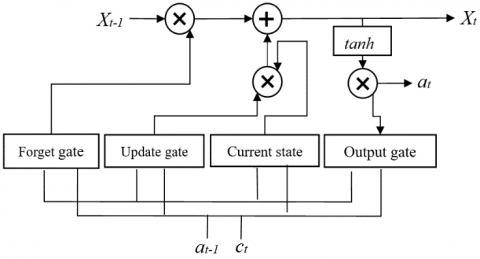Figure 1. The structure of an LSTM unit

As shown in (4) and (5), the unit state Xt-1 is updated into Xt. The cell could retain or update the current state, using the update gate and the forget gate. Like other DL algorithms, the LSTM network obtains the optimal solution by error backpropagation.

When it comes to insurance sales prediction, it is difficult to fit the sales trend of all kinds of insurances, because the original sales data involve various insurances and change nonlinearly with time. To overcome the difficulty, this paper decides to design an insurance sales prediction model that couples LINEST function-based MLR, gray correlation analysis, and LSTM network, aiming to eliminate the redundancy of network inputs, speed up the DL convergence of LSTM algorithm, and improve prediction accuracy.

The LINEST function-based MLR can effectively clarify the correlations between source data, prevent the local minimum trap, and optimize the prediction model with a limited number of source data. Meanwhile, the gray correlation analysis can eliminate the nonlinear influence of various factors (e.g. holidays, seasons, online promotions, and offline promotions) on the prediction model, and thus improve the accuracy and convergence speed of the model.

As shown in Figure 2, the insurance sales prediction model was constructed in the following steps:

Step 1. Create an original database based on the historical data of insurance sales, identify the linear and nonlinear factors (number of online and offline promotions, holiday distribution, and seasonal sales index) affecting the sales, and construct the cause-and-effect diagram and decision matrix:

$A=\frac{{\sum\limits_{i=0}^{n}{{{m}_{i}}}}/{n}\;}{{\sum\limits_{j-0}^{12}{{{n}_{j}}}}/{12}\;}$         (7)

where, mi is the sales in the i-th year out of n years; ni is the sales in the j-th month of the i-th year.

Step 2. Nondimensionalize the data, compute and compare the gray correlations of different factors, and make comprehensive evaluation of insurance marketing based on the correlations.

Step 3. Perform MLR on the data using the LINEST function, establish data links between units, define formulas and set up the MLR model, derive the coefficient of multiple determinations, and remove the redundant factors.

Step 4. Predict and optimize residual functions repeatedly to improve the convergence speed and prediction effect of LSTM network, and introduce the decision matrix, which has gone through nondimensionalization and gray correlation calculation, into the LSTM network to predict the insurance sales.

## 2.png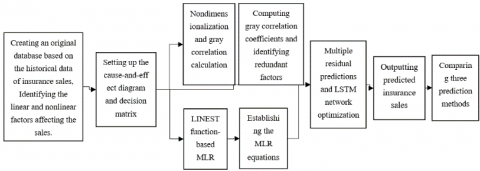Figure 2. The flow of model construction

3. Model Solving

3.1 Correlation analysis

In actual insurance sales prediction, the forecasted trend is resulted from the combined effects of multiple factors. The predicted result varies with the degree of impact from the various factors. Many algorithms have been developed to process the main influencing factors quickly and effectively, including principal component analysis (PCA), mean squared error (MSE) analysis, and regression analysis. However, these algorithms are not applicable unless the data obey the probabilistic distribution, and are complex in computation. In the case of limited data, the results predicted by these algorithms tend to deviate from the actual data. To solve the problem, the gray correlation analysis was introduced to analyze the original data.

Insurance sales prediction is affected by nonlinear factors like holidays, seasons, online promotions, and offline promotions. Considering the numerous targets and complex bases of the decision matrix, the historical data on insurance sales were preprocessed through gray correlation analysis.

Firstly, the reference sequence X0 for insurance sales was determined, and m comparison sequences X1, …, Xm were established, including the historical sales, number of online promotions, number of offline promotions, holiday distribution, and seasonal sales index. The optimal reference value was selected against evaluation indices.

Secondly, the reference sequence and comparison sequences were nondimensionalized. Through average transform, the correlation matrix can be expressed as:

${{\left( {{X}_{0}},{{X}_{1}},\cdots ,{{X}_{m}} \right)}^{T}}=\left[ \begin{matrix} {{X}_{10}} & {{X}_{20}} & \cdots & {{X}_{n0}} \\ {{X}_{11}} & {{X}_{21}} & \cdots & {{X}_{n1}} \\ \vdots & \vdots & \vdots & \vdots \\ {{X}_{1m}} & {{X}_{2m}} & \cdots & {{X}_{nm}} \\\end{matrix} \right]\text{ }$       (8)

(3) The second-order minimum and maximum ranges were calculated row by row between reference sequence and comparison sequences, and the gray correlation coefficients were computed by:

${{\zeta }_{i}}j=\frac{\underset{i}{\mathop{\min }}\,\underset{j}{\mathop{\min }}\,\left| {{X}_{i0}}-{{X}_{ij}} \right|+\rho \underset{i}{\mathop{\max }}\,\underset{j}{\mathop{\max }}\,\left| {{X}_{i0}}-{{X}_{ij}} \right|}{\left| {{X}_{i0}}-{{X}_{ij}} \right|+\rho \underset{i}{\mathop{\max }}\,\underset{j}{\mathop{\max }}\,\left| {{X}_{i0}}-{{X}_{ij}} \right|}$        (9)

(4) According to the calculated correlation coefficients of i=1, 2, ...n and j=1, 2, ...m, the gray correlations between the reference sequence X0 and each comparison sequence, and between comparison sequences can be obtained as:

${{g}_{i}}=\frac{1}{n}\sum\limits_{j=1}{{{\zeta }_{i}}(j)}\text{ }$        (10)

(5) The gray correlation coefficients were ranked by gray correlations. The ranking reflects the degree of impact from each factor on the prediction effect. The weakly correlated factors and their data were removed.

3.2 The MLR

The processing of insurance sales data should not impose special requirements on the data size. Otherwise, it is impossible to build a model with good prediction effect under limited data resources.

Here, the data from insurance sales database are linearly fitted by least squares (LS) method, using the LINEST function. In this way, the prediction model can be established simultaneously with the correlation analysis on the data variation under different factors, while simplifying the data structure.

The reference sequence of insurance sales can be defined as:

$X_{0}^{0}=\{X_{10}^{0},X_{20}^{0},\ldots X_{i0}^{0},\ldots X_{n0}^{0}\}$          (11)

The comparison sequences can be established as:

\begin{align} & X_{1}^{0}=\{X_{11}^{0},X_{21}^{0},\ldots X_{i1}^{0},\ldots X_{n1}^{0}\} \\ & X_{2}^{0}=\{X_{12}^{0},X_{22}^{0},\ldots X_{i2}^{0},\ldots X_{n2}^{0}\} \\ & \text{ }\vdots \\ & X_{m}^{0}=\{X_{1m}^{0},X_{2m}^{0},\ldots X_{im}^{0},\ldots X_{nm}^{0}\} \\ \end{align}         (12)

The above sequences were added into the updated sequence:

$X_{i}^{1}=\left\{ X_{ji}^{1}|X_{ji}^{1}=\sum\limits_{k=1}^{j}{X_{ki}^{1},j=1,2,\ldots ,m} \right\}$          (13)

where, j=1, 2, ...m. The gray differential function for (13) can be expressed as:

$\frac{dX_{t1}^{1}}{dt}+{{b}_{1}}X_{t1}^{1}={{b}_{2}}X_{t2}^{1}+\ldots +{{b}_{m}}X_{tm}^{1}\text{ }$        (14)

where, t=1, 2, ...n. Then, the updated sequence was estimated by LINEST function:

${{\left[ {{b}_{1}},{{b}_{2}},\ldots {{b}_{n}} \right]}^{T}}={{({{B}^{T}}B)}^{-1}}{{B}^{T}}{{W}_{R}}$          (15)

where,

B=\left[ \begin{align} & -\frac{X_{11}^{1}+X_{21}^{1}}{2}\text{ }X_{22}^{1}\text{ }X_{23}^{1}\text{ }\ldots \text{ }X_{2m}^{1} \\ & -\frac{X_{21}^{1}+X_{31}^{1}}{2}\text{ }X_{32}^{1}\text{ }X_{33}^{1}\text{ }\ldots \text{ }X_{3m}^{1} \\ & \text{ }\cdots \text{ }\cdots \text{ }\cdots \text{ }\cdots \text{ }\cdots \text{ } \\ & -\frac{X_{(n-1)1}^{1}+X_{n1}^{1}}{2}\text{ }X_{n2}^{1}\text{ }X_{n3}^{1}\text{ }\ldots \text{ }X_{nm}^{1} \\ \end{align} \right]          (16)

${{W}_{R}}={{\left[ X_{21}^{0},X_{31}^{0},\ldots ,X_{n1}^{0} \right]}^{T}}$          (17)

Hence, the future insurance sales can be estimated by:

$\hat{X}_{t1}^{0}=\left( \hat{X}_{11}^{0}-\frac{1}{{{b}_{1}}}\sum\limits_{i=2}^{n}{{{b}_{i}}\hat{X}_{ti}^{0}} \right)\cdot {{e}^{{{b}_{1}}(t-1)}}+\frac{1}{{{b}_{1}}}\sum\limits_{i=2}^{n}{{{b}_{i}}\hat{X}_{ti}^{0}}$         (18)

where, t gradually changes from 2 to the sum of n and the number of items to be predicted.

3.3 Solving the LSTM network

Based on the features of insurance sales prediction, the main influencing factors of insurance sales were identified, in the light of the gray correlations. Then, the data structure was simplified through the MLR. The weakly correlated factors were removed, and the remaining factors were imported to the LSTM network to predict the insurance sales. Thus, the predicted value of the updated sequence was obtained. During the solving process, multiple residual predictions were conducted to optimize the LSTM network, thereby improving the learning rate. The specific solving process is as follows:

Step 1. Predict the residual sequence ε between the reference sequence and comparison sequences through the BPNN of the LSTM network, and denote the predicted result as ε'.

Step 2. Since the historical trend could affect the future trend of insurance sales, compute the difference sequence between the residual sequence ε, which contains historical sales data, and the predicted residual ε'; take the difference sequence as the comparison sequence, perform BPNN prediction in the LSTM network, and denote the predicted result as ε''.

Step 3. Add the predicted residuals in Steps 1 and 2 into ε''', and add ε''' with the result of (18) into the predicted sequence.

For the lack of space, only two residual predictions are mentioned above. In actual solving process, more residual predictions must be carried out. Since insurance sales prediction is affected by various linear and nonlinear factors, the actual prediction must ensure that each factor reasonably goes through residual prediction, making the predicted result more reliable.

4. Model Training and Testing

4.1 Data preprocessing

The original data are the four-year billing data (January 2016-December 2019) of a well-known insurance company. Here, the original data are divided into 207 kinds of insurances in 18 classes, including 10 property insurances (i.e. corporate property insurance, engineering insurance, auto insurance, liability insurance, ship insurance, cargo insurance, home property insurance, credit insurance, guarantee insurance, and agricultural insurance) and 8 personal insurances (i.e. accident insurance, medical insurance, critical illness insurance, life insurance, children’s education insurance, pension insurance, annuity insurance, and group insurance).

The daily sales of each insurance were counted and analyzed preliminarily. It is learned that the sales data of some insurances are missing. If the original database is directly used for sales prediction, the missing values should be padded, or filled with means or medians. The most desirable method is the proposed MLR based on date features: the missing data were combined with the annual sales index, and the dates of missing data were processed as those in other years.

Outliers refer to the data that deviate greatly from most data in the original database. During insurance sales prediction, the outliers must be identified and eliminated. Here, an insurance sales data sequence is considered as an outlier, if the difference of the sequence and the mean of insurance sales data sequences in the same period is more than 3 times the standard deviation of such sequences.

## 3.png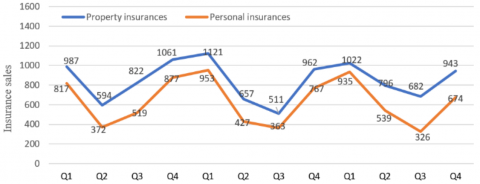Figure 3. The periodical trend of insurance sales

As shown in Figure 3, the seasonal insurance sales data obey a sinusoidal distribution, with the first quarter (Q1) and the fourth quarter (Q4) as the start and end of a cycle, respectively. The insurance sales data have seasonal similarities: the sales in Q1 and Q4 were higher than those in Q2 and Q3. As a result, the sales data of three years from the original database were divided into 12 seasonal data sequences.

4.2 Model training

Based on the original database, the hot sales of each kind of insurance were summarized. Then, the data were divided by season as per the date features of insurance sales. The data on holidays were highlighted in the decision matrix.

Concerning the training algorithm, the predicted values of the proposed DL-based LSTM network were used to predict residuals through multiple backpropagations. During the residual predictions, the state in the previous moment was iteratively updated. The current state was substituted into the loss function to obtain the current gradient. The iterations continued until the algorithm reached convergence.

To achieve ideal prediction, the training set was created based on the insurance sales data in 2016-2018, and the test set was developed based on the data in 2019. Figure 4 provides the loss curve of our model in the training process.

## 4.png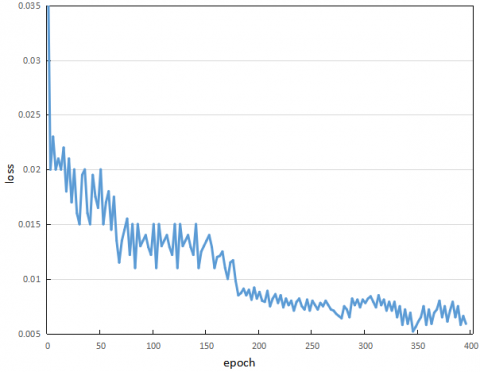Figure 4. The loss curve of model training

4.3 Sales prediction

The previous sections theoretically explore the feasibility of applying the LSTM network to insurance sales prediction, introduce the principles of building the insurance sales prediction model, and illustrate the workflow of model solving. This sub-section intends to analyze the prediction error of our method through simulation, and compare the performance of our model with other sales prediction models.

## 5.png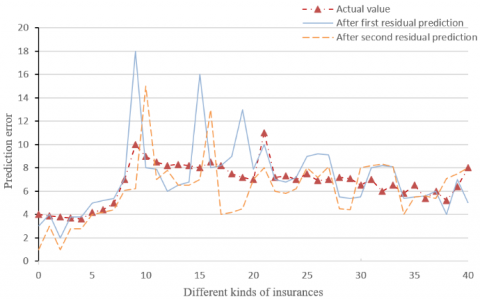Figure 5. The predicted results after residual predictions

## 6.png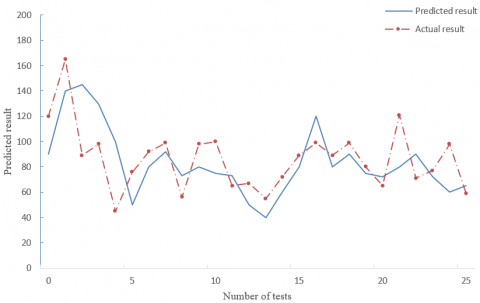Figure 6. The results predicted by traditional LSTM network

## 7.png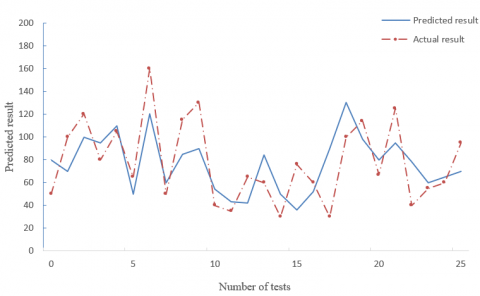Figure 7. The results predicted by GA-optimized BPNN

## 8.png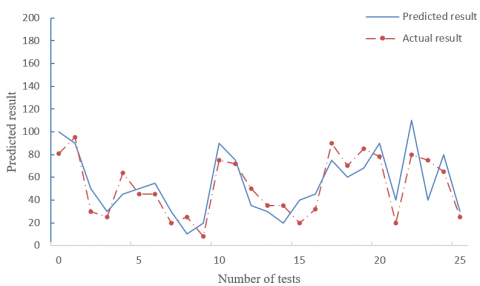Figure 8. The results predicted by our model

## 9.png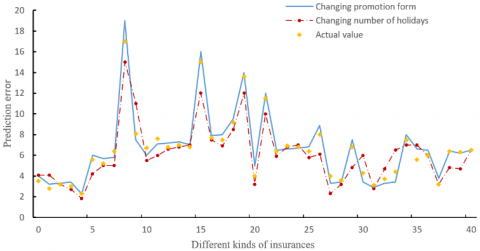Figure 9. The seasonable sales of each insurance predicted by our model

Figure 5 compares the predicted results of our model before and after two residual predictions with the actual values. Obviously, the theoretical prediction fully agrees with the actual values. After the first residual prediction, the predicted results of 40 insurances lagged behind the actual values, which could not be mitigated by changing the bias values. However, the predicted results were desirable after the second residual prediction.

Next, the LSTM network processed by LINEST function-based MLR (our model) was compared with the traditional LSTM network and the GA-optimized BPNN in the prediction of insurance sales. The predicted results of the three models are displayed in Figures 6-8.

Comparing Figures 6 and 8, it is learned that the GA-optimized BPNN predicted the insurance sales well, when the seasonal sales were in stable or peak state, and fitted the sales trend accurately. With the growing number of tests, the result predicted by our model was closer to the actual result in 2019 than that predicted by the GA-optimized BPNN, and the error rate of our model declined from 26.77% to 8.25%.

Comparing Figures 7 and 8, it is learned that the traditional LSTM network could not predict insurance sales accurately, when the reference sequence had great fluctuations. This means the traditional LSTM network is unable to adapt to the sales fluctuations induced by sudden factors. By contrast, our model achieved a good prediction effect, by processing the data sequences through MLR, and reduced the error rate by 12.5%.

Figure 9 presents the seasonable sales of each insurance predicted by our model. It can be seen that our model fitted the sales trend well, even if the promotion form and the number of holidays were changed. In particular, the mean error was controlled at 16 items/week, when the seasonal sales remained stable. Of course, there is still room to improve the prediction accuracy at some time points, where the sales suddenly increased or decreased.

5. Conclusions

This paper mainly improves the LSTM network and designs an insurance sales prediction model. Firstly, an original database was created on the historical data of insurance sales. Then, the factors affecting insurance sales, namely, promotions, holidays, and seasons, were determined, and the cause-and-effect diagram and decision matrix were established. Next, the redundant data were removed from data sequences through nondimensionalization and gray correlation calculation. Finally, the insurance sales were predicted through the LINEST function-based MLR. Through simulations, the proposed model was found to outperform the traditional LSTM network and the GA-optimized BPNN in insurance sales prediction. This means the prediction effect can be improved by data processing through multiple residual predictions.

Acknowledgment

This research was financially supported by Ministry of Education of China Youth Fund Project of Humanities and Social Sciences (Grant No.: 13YJC630162), 2018 Annual project of China Insurance Society (Grant No.: ISCKT2018-N-1-14) and by abroad visiting scholar program of Shandong University of Finance and Economics.

References

 Ren, S., Chan, H.L., Ram, P. (2017). A comparative study on fashion demand forecasting models with multiple sources of uncertainty. Annals of Operations Research, 257(1-2): 335-355. https://doi.org/10.1007/s10479-016-2204-6

 Van Calster, T., Baesens, B., Lemahieu, W. (2017). ProfARIMA: A profit-driven order identification algorithm for ARIMA models in sales forecasting. Applied Soft Computing, 60: 775-785. https://doi.org/10.1016/j.asoc.2017.02.011

 Aljawarneh, S., Aldwairi, M., Yassein, M.B. (2018). Anomaly-based intrusion detection system through feature selection analysis and building hybrid efficient model. Journal of Computational Science, 25: 152-160. https://doi.org/10.1016/j.jocs.2017.03.006

 Fan, Z.P., Che, Y.J., Chen, Z.Y. (2017). Product sales forecasting using online reviews and historical sales data: A method combining the Bass model and sentiment analysis. Journal of Business Research, 74: 90-100. https://doi.org/10.1016/j.jbusres.2017.01.010

 Raja, M.C., Rabbani, M.M.A. (2016). Combined analysis of support vector machine and principle component analysis for IDS. In 2016 International Conference on Communication and Electronics Systems (ICCES), pp. 1-5. https://doi.org/10.1109/CESYS.2016.7889868

 He, K., Zhang, X., Ren, S., Sun, J. (2016). Deep residual learning for image recognition. In Proceedings of the IEEE Conference on Computer Vision and Pattern Recognition, pp. 770-778. https://doi.org/10.1109/CVPR.2016.90

 Abdel-Zaher, A. M., Eldeib, A.M. (2016). Breast cancer classification using deep belief networks. Expert Systems with Applications, 46: 139-144. https://doi.org/10.1016/j.eswa.2015.10.015

 Almansob, S.M., Lomte, S.S. (2017). Addressing challenges for intrusion detection system using naive Bayes and PCA algorithm. In 2017 2nd International Conference for Convergence in Technology (I2CT), pp. 565-568. https://doi.org/10.1109/I2CT.2017.8226193

 Sharafaldin, I., Lashkari, A. H., Ghorbani, A. A. (2018). Toward generating a new intrusion detection dataset and intrusion traffic characterization. In ICISSP, pp. 108-116. https://doi.org/10.5220/0006639801080116

 Park, H.D., Min, O.G., Lee, Y.J. (2017). Scalable architecture for an automated surveillance system using edge computing. The Journal of Supercomputing, 73(3): 926-939. https://doi.org/10.1007/s11227-016-1750-7

 Sun, L., Anthony, T.S., Xia, H.Z., Chen, J., Huang, X., Zhang, Y. (2017). Detection and classification of malicious patterns in network traffic using Benford's law. In 2017 Asia-Pacific Signal and Information Processing Association Annual Summit and Conference (APSIPA ASC), pp. 864-872. https://doi.org/10.1109/APSIPA.2017.8282154

 Cheng, G., Han, J., Lu, X. (2017). Remote sensing image scene classification: Benchmark and state of the art. Proceedings of the IEEE, 105(10): 1865-1883. https://doi.org/10.1109/JPROC.2017.2675998

 Ibrahimi, K., Ouaddane, M. (2017). Management of intrusion detection systems based-KDD99: Analysis with LDA and PCA. In 2017 International Conference on Wireless Networks and Mobile Communications (WINCOM), pp. 1-6. https://doi.org/10.1109/WINCOM.2017.8238171

 Marir, N., Wang, H., Feng, G., Li, B., Jia, M. (2018). Distributed abnormal behavior detection approach based on deep belief network and ensemble SVM using spark. IEEE Access, 6: 59657-59671. https://doi.org/10.1109/ACCESS.2018.2875045

 Cai, J., Wohn, D.Y. (2019). Live streaming commerce: Uses and gratifications approach to understanding consumers’ motivations. In Proceedings of the 52nd Hawaii International Conference on System Sciences, ISBN: 978-0-9981331-2-6. https://doi.org/10.24251/HICSS.2019.307

 Fadhil, A.F., Kanneganti, R., Gupta, L., Eberle, H., Vaidyanathan, R. (2019). Fusion of enhanced and synthetic vision system images for runway and horizon detection. Sensors, 19(17): 3802. https://doi.org/10.3390/s19173802

 Wazwaz, A.A., Herbawi, A.O., Teeti, M.J., Hmeed, S.Y. (2018). Raspberry Pi and computers-based face detection and recognition system. In 2018 4th International Conference on Computer and Technology Applications (ICCTA), pp. 171-174. https://doi.org/10.1109/CATA.2018.8398677

 Guan, Z.Y., Li, J., Yang, H. (2016). Runway extraction method based on rotating projection for UAV. In Proceedings of the 6th International Asia Conference on Industrial Engineering and Management Innovation, pp. 311-324. https://doi.org/10.2991/978-94-6239-145-1_30

 Nalepa, J., Kawulok, M. (2019). Selecting training sets for support vector machines: A review. Artificial Intelligence Review, 52(2): 857-900. https://doi.org/10.1007/s10462-017-9611-1

 Aksu, D., Üstebay, S., Aydin, M.A., Atmaca, T. (2018). Intrusion detection with comparative analysis of supervised learning techniques and fisher score feature selection algorithm. In International Symposium on Computer and Information Sciences, pp. 141-149. https://doi.org/10.1007/978-3-030-00840-6_16

 Sharafaldin, I., Lashkari, A.H., Ghorbani, A.A. (2018). Toward generating a new intrusion detection dataset and intrusion traffic characterization. In ICISSP, pp. 108-116. https://doi.org/10.5220/0006639801080116

 Resende, P.A.A., Drummond, A.C. (2018). Adaptive anomaly‐based intrusion detection system using genetic algorithm and profiling. Security and Privacy, 1(4): e36. https://doi.org/10.1002/spy2.36

 Chen, F., Ren, R., Van de Voorde, T., Xu, W., Zhou, G., Zhou, Y. (2018). Fast automatic airport detection in remote sensing images using convolutional neural networks. Remote Sensing, 10(3): 443. https://doi.org/10.3390/rs10030443

 Cai, B., Jiang, Z., Zhang, H., Zhao, D., Yao, Y. (2017). Airport detection using end-to-end convolutional neural network with hard example mining. Remote Sensing, 9(11): 1198. https://doi.org/10.3390/rs9111198